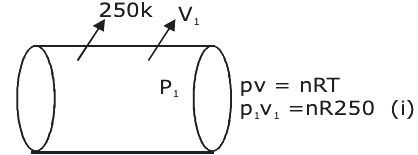Checkout JEE MAINS 2022 Question Paper Analysis : Checkout JEE MAINS 2022 Question Paper Analysis :

# Initially a gas of diatomic molecules are contained in a cylinder

Initially a gas of diatomic molecules are contained in a cylinder of volume V1 at a pressure P1 and temperature 250 K. Assuming that 25% of the molecules get dissociated causing a change in the number of moles. The pressure of the resulting gas at temperature 2000 K, when contained in a volume 2V1 is given by P2. The ratio P2 / P1 is ________.25% will dissociate out of 100.

3n / 4 molecules will remain the same as S (n / 4) mole become → n / 2

∴ Total molecules used

→ (3n / 4) + (n / 2) = (5n / 4)

P22V1 = (5n / 4) . R . 2000 — (ii)

Equation (ii / i)

2p2v1 / p1v1 = [5nR × 2000] / [4nR × 250]

(P2 / P1) = 5(11)(10)